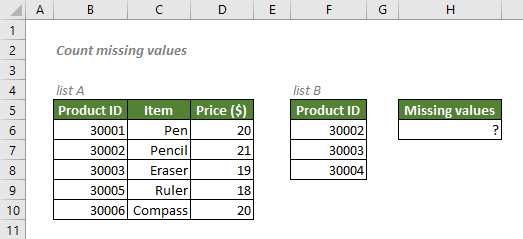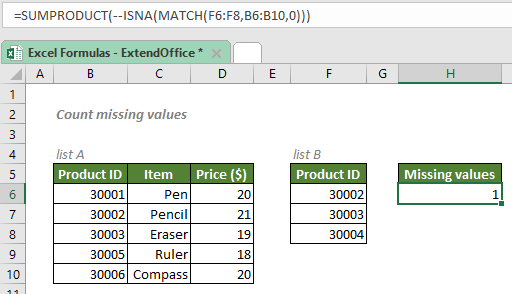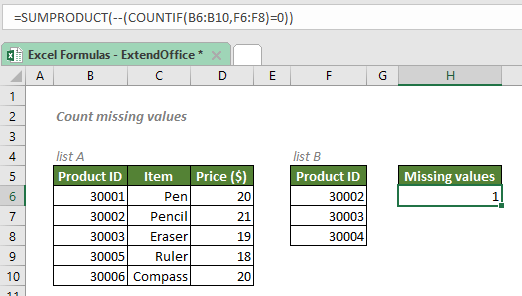## Count missing values

Supposing you have two lists, to count the total number of values in one list that do not exist in another list, you can use a SUMPRODUCT formula with the help of MATCH and ISNA functions, or the COUNTIF function.#### Count missing values with SUMPRODUCT, MATCH and ISNA

To count the total number of values in list B that are missing from list A as shown above, you can first use the MATCH function to return an array of the relative position of the values from list B in the list A. If a value does not exist in list A, an #N/A error will be returned. The ISNA function will then identify the #N/A errors and SUMPRODUCT will count the total number of the errors.

#### Generic syntax

=SUMPRODUCT(--ISNA(MATCH(range_to_count,lookup_range,0)))

• range_to_count: The range from which to count missing values. Here refers to the list B.
• lookup_range: The range to compare with the range_to_count. Here refers to the list A.
• 0: The match_type 0 forces MATCH to perform an exact match.

To count the total number of values in list B that are missing from list A, please copy or enter the formula below in the cell H6, and press Enter to get the result:

=SUMPRODUCT(--ISNA(MATCH(F6:F8,B6:B10,0)))#### Explanation of the formula

=SUMPRODUCT(--ISNA(MATCH(F6:F8,B6:B10,0)))

• MATCH(F6:F8,B6:B10,0): The match_type 0 forces the MATCH function to return numeric values that indicate the relative positions of the values through cells F6 to F8 in the range B6:B10. If a value does not exist in list A, an #N/A error will be returned. So, the results will be in an array like this: {2;3;#N/A}.
• ISNA(MATCH(F6:F8,B6:B10,0)) = ISNA({2;3;#N/A}): ISNA works to find out whether a value is an “#N/A” error or not. If yes, the function will return a TURE; If not, it will return a FALSE. So, the ISNA formula will return {FALSE;FALSE;TRUE}.
• SUMPRODUCT(--ISNA(MATCH(F6:F8,B6:B10,0))) = SUMPRODUCT(--{FALSE;FALSE;TRUE}): The double negative sign converts TRUEs to 1s and FALSEs to 0s: {0;1;0}. Then the SUMPRODUCT function returns the sum: 1.

#### Count missing values with SUMPRODUCT and COUNTIF

To count the total number of values in list B that are missing from list A, you can also use the COUNTIF function to tell if a value exists in list A or not with the condition “=0”, since a 0 will generate if a value is missing. The SUMPRODUCT will then count the total number of missing values.

#### Generic syntax

=SUMPRODUCT(--(COUNTIF(lookup_range,range_to_count)=0))

• lookup_range: The range to compare with the range_to_count. Here refers to the list A.
• range_to_count: The range from which to count missing values. Here refers to the list B.
• 0: The match_type 0 forces MATCH to perform an exact match.

To count the total number of values in list B that are missing from list A, please copy or enter the formula below in the cell H6, and press Enter to get the result:

=SUMPRODUCT(--(COUNTIF(B6:B10,F6:F8)=0))#### Explanation of the formula

=SUMPRODUCT(--(COUNTIF(B6:B10,F6:F8)=0))

• COUNTIF(B6:B10,F6:F8): The COUNTIF function counts the occurrence number of the cells through F6 to F8 in the range B6:B10. The result will be in an array like this: {1;1;0}.
• --(COUNTIF(B6:B10,F6:F8)=0) = --({1;1;0}=0): The snippet {1;1;0}=0 yields a TRUE and FALSE array {FALSE;FALSE;TRUE}. The double negative sign then turns TRUEs to 1s and FALSEs to 0s. The final array is like this: {0;0;1}.
• SUMPRODUCT(--(COUNTIF(B6:B10,F6:F8)=0)) = SUMPRODUCT({0;0;1}): The SUMPRODUCT then returns the sum: 1.

#### Related functions

Excel SUMPRODUCT function

In Excel, the SUMPRODUCT function can be used to multiply two or more columns or arrays together, and then get the sum of products. In fact, the SUMPRODUCT is a useful function which can help to count or sum cell values with multiple criteria like the COUNTIFS or SUMIFS function. This article will introduce the function syntax and some examples for this SUMPRODUCT function.

Excel MATCH function

The Excel MATCH function searches for a specific value in a range of cells, and returns the relative position of the value.

Excel COUNTIF function

The COUNTIF function is a statistical function in Excel which is used to count the number of cells that meet a criterion. It supports logical operators (<>, =, >, and <), and the wildcards (? and *) for partial matching.

#### Related Formulas

Find missing values

There are cases when you need to compare two lists to check if a value of list A exists in list B in Excel. For example, you have a list of products, and you want to check if the products in your list exist in the product list provided by your supplier. To accomplish this task, here we listed three ways below, feel free to pick the one you like.

Count cells equal to

This article will focus on the Excel formulas for counting the cells which are exactly equal to a text string you specify or partial equal to the given text string as below screenshots shown. Firstly, it will explain the formula syntax and argument, the provides examples for you to better understand.

Count number of cells not between two given numbers

To count the number of cells between two numbers is a common task for us in Excel, but, in certain case, you may want to count the cells not between two given numbers. For example, I have a product list with the sales from Monday to Sunday, now, I need to get the number of cells which not between the specific low and high numbers as below screenshot shown. This article, I will introduce some formulas for dealing with this task in Excel.

### The Best Office Productivity Tools

#### Kutools for Excel - Helps You To Stand Out From Crowd

Would you like to complete your daily work quickly and perfectly? Kutools for Excel brings 300 powerful advanced features (Combine workbooks, sum by color, split cell contents, convert date, and so on...) and save 80% time for you.

• Designed for 1500 work scenarios, helps you solve 80% Excel problems.
• Reduce thousands of keyboard and mouse clicks every day, relieve your tired eyes and hands.
• Become an Excel expert in 3 minutes. No longer need to remember any painful formulas and VBA codes.
• 30-day unlimited free trial. 60-day money back guarantee. Free upgrade and support for 2 years.#### Office Tab - Enable Tabbed Reading and Editing in Microsoft Office (include Excel)

• One second to switch between dozens of open documents!
• Reduce hundreds of mouse clicks for you every day, say goodbye to mouse hand.
• Increases your productivity by 50% when viewing and editing multiple documents.
• Brings Efficient Tabs to Office (include Excel), Just Like Chrome, Firefox, And New Internet Explorer.